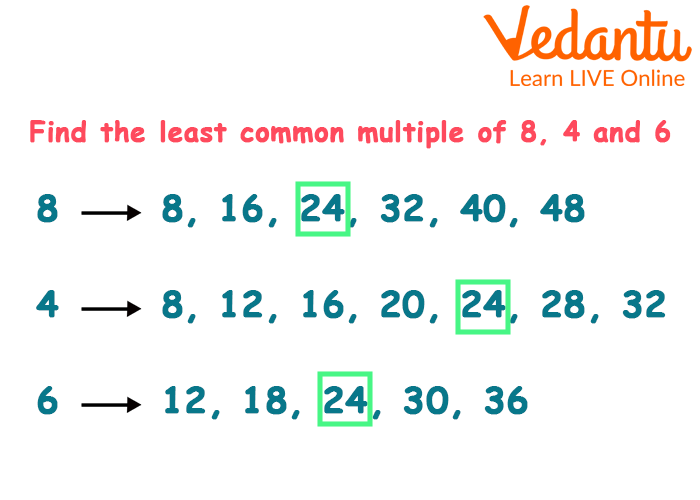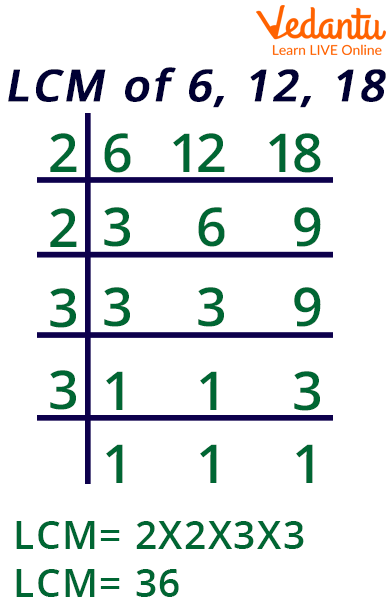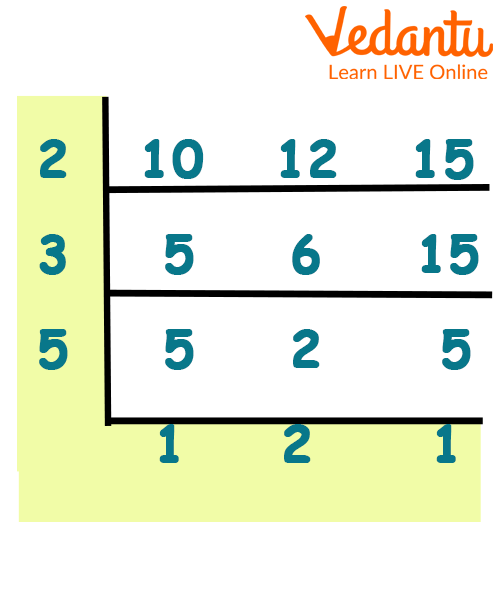Courses
Courses for Kids
Free study material
Free LIVE classes
More

# LCM with 3 NumbersLIVE
Join Vedantu’s FREE Mastercalss

## Introduction to LCM

The least common multiple (LCM) is the smallest positive integer that is divided by both a and b. Its official name is Least Common Multiple.

The LCM method is used to determine the least multiple of two or more numbers. Both of the numbers will divide the LCM. When performing any arithmetic operations involving fractions, such as addition and subtraction, LCM is utilized to make the denominators common. This method facilitates the simplification process.

### What is LCM?

When the denominators of two fractions are different, LCM can also be used to add or subtract the fractions. The smallest positive integer that is divided by both a and b is known as the least common multiple (LCM) .A number that is a multiple of two or more other numbers is said to be a common multiple.

### LCM with 3 Numbers:

LCM is represented as LCM for three integers a, b, and c. For instance, the smallest number that can be divided by all three integers is 60, which is the LCM of 12, 15, and 10. ${\rm{LCM}}\left[ {12,15,10} \right]$ thus equals 60.

### How to Calculate LCM of Three Numbers:

To find 3 Numbers LCM with Listing Multiples:

This is a very interesting process to How to Take the LCM of 3 Numbers:

• List every multiple of three numbers until at least one of them appears on every list.

• Find the number that appears on all of the lists and is the least.LCM of 3 Numbers Formula(for 8, 4, 6)

### Utilizing Prime Factorization, get the LCM of Three Numbers:

• Make a list of each of the provided numbers' prime factors.

• List all the prime numbers you've discovered, in order of how frequently they appear in the given numbers.

• To find the Least Common Multiple, multiply the list of prime numbers.LCM of 6,12,18

### Find LCM of Numbers Using Cake/ Ladder Method:

• Put the three numbers in a row or cake pattern.

• Bring the result into the following layer after dividing the integers in the layer by the given number that is equally divisible by all those numbers present.

• Simply bring any non-divisible number down if it is present in the layer or row.

• Divide the rows by prime numbers once more.

• You are finished when there are no more numbers.

• Then, multiply all the numbers together and you will get the LCM of required numbers.

### Properties of LCM:

PROPERTY 1 : LCM obeys Associative Property

${\rm{LCM}}\left[ {c,d} \right] = {\rm{LCM}}\left[ {d,c} \right]$

PROPERTY 2 : LCM obeys Commutative Property

${\rm{LCM}}\left[ {a,b,c} \right] = {\rm{LCM}}\left[ {{\rm{LCM}}\left[ {a,b} \right],c} \right] = {\rm{LCM}}\left[ {a,{\rm{LCM}}\left[ {b,c} \right]} \right]$

PROPERTY 3 : LCM obeys Distributive Property

${\rm{LCM}}\left[ {da,db,dc} \right] = d \times {\rm{LCM}}\left[ {a,b,c} \right]$

### Conclusion

LCM can be used to add or subtract two fractions when their respective denominators are different. The least common multiple (LCM) is the smallest positive integer that is divided by both a and b. (LCM).

### Solved Example :

Example 1 : Find ${\rm{LCM}}\left[ {6,7,21} \right]$ by listing Multiples.

Solution : Given digits 6, 7, and 21

There are six multiples: 6, 12, 18, 24, 30, 36, 42, 48, 54, and 60

7 is a multiple of 14, 21, 28, 35, 42, 49, and 56.

21 is a multiple of 21, 42, and 63.

According to the definition, the least common multiple [LCM] of all three integers is the smallest number.

Thus, the LCM of 6, 7, and 21 is 42.

Example 2 : Find LCM of 12, 24, 30 using Prime Factorization Method?

Solution : 12's prime factorization is $2 \times 2 \times 3$

24's prime factorization is $2 \times 2 \times 2 \times 2 \times 3$

prime factorization of 30 will be $2 \times 3 \times 5$

Make a note of the often occurring prime numbers for the provided numbers, then multiply them.

$= 2 \times 2 \times 2 \times 3 \times 5$

$= 120$

Thus, its LCM of given numbers is 120.

Example 3 : Find the LCM(10, 12, 15) using the Cake/ Ladder Method.

Solution : Numbers given are 10, 12, and 15.LCM of 10,12,15 by Cake/Ladder Method

To locate LCM From top to bottom, multiply all the prime integers, i.e.

$2 \times 3 \times 5 \times 1 \times 2 \times 1 = 60$

LCM(10, 12, 15) is therefore 60.

Last updated date: 18th Sep 2023
Total views: 79.5k
Views today: 1.79k

## FAQs on LCM with 3 Numbers

1.How to determine a number's LCM?

There are three main ways to get the LCM of a set of numbers: by listing the multiples of the provided numbers, by factoring the numbers into their prime factors, and by cake or ladder method.

2.What are 4 and 5's LCM?

20 is the least frequent multiple of 4 and 5.

3. What is LCM?

The LCM method is used to determine the least multiple of two or more numbers. Both of the numbers will divide the LCM.

4.Find the smallest number that is precisely divisible by 3, 9, or 18.

Their least common multiple (LCM) is the lowest number that can be divided exactly by 3, 9, and 18.

3 is a multiple of 6, 9, 12, 15, 18, 21, etc.

9 is a multiple of 18, 27, 36, 45, 54, 63, etc.

18 divided by 18 equals 18, 36, 54, 72, 90, 108, 126, etc.

The LCM of 3, 9, and 18 is hence 18.

5.The LCM of 12 and 8 is what?

Write the multiples of 12 and 8 first.

12 times: 12, 24, 36, etc.

Numerous 8s include 8, 16, 24, 32, etc.

The least common multiple is 24, as may be seen. Because of this, the LCM of $\left[ {12,8} \right]$ is 24.